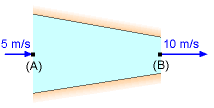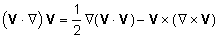Ch 5. Fundamental Laws (Differential) Multimedia Engineering Fluids ConservationMass Navier-Stokesand Euler's ConservationEnergy
 Chapter 1. Basics 2. Fluid Statics 3. Kinematics 4. Laws (Integral) 5. Laws (Diff.) 6. Modeling/Similitude 7. Inviscid 8. Viscous 9. External Flow 10. Open-Channel Appendix Basic Math Units Basic Equations Water/Air Tables Sections Search eBooks Dynamics Fluids Math Mechanics Statics Thermodynamics Author(s): Chean Chin Ngo Kurt Gramoll ©Kurt GramollFLUID MECHANICS - CASE STUDY IntroductionProblem Description Kenneth is planning to enter the doctoral program, and he needs to pass the qualifying exam to be admitted. One question in the recent qualifying exam is from fundamental fluid mechanics. The first part is to derive Bernoulli's equation from Euler's equation. The second part is to use the derived Bernoulli's equation to predict the pressure drop across a nozzle, as shown in the figure. The nozzle is used to accelerate and double the air velocity. Questions (a) Derive Bernoulli's equation from Euler's equation. (b) What is the pressure difference between the inlet and outlet of the nozzle? Approach Begin the derivation process with Euler's equation in vector form. Use the following vector identity:Assume the change in the elevation is negligible when determining the pressure drop, i.e., a horizontal nozzle. The density of air is 1.23 kg/m3.

Practice Homework and Test problems now available in the 'Eng Fluids' mobile app
Includes over 250 problems with complete detailed solutions.
Available now at the Google Play Store and Apple App Store.